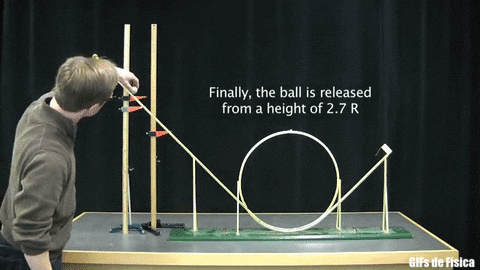Motion in Vertical Circle↪ Suppose an object of mass m tied at one end of a string is whirling in a vertical circle of radius r and center O.
↪ The object is moving with a uniform velocity v.⁕ Object at point A
↪ When the object arrives at point A, its weight mg and the tension TA in the string act in the opposite direction.
↪ Therefore the force toward the center O is↪ This is a maximum tension in the string.
↪ Thus tension is a maximum when the object is at the lowest point of the vertical circle.

⁕ Object at point B or B'
↪ When the object arrives at point B or at point B', its weight mg acts at right angles to the tension TB or TB' in the string.
↪ Therefore the force toward the center O is↪ Thus the centripetal force is equal to the tension in the string.

⁕ Object at point C
↪ When the object arrives at point C, its weight mg and the tension TC both act in the same direction toward the center.
↪ Therefore the force toward the center O is↪ This is a minimum tension in the string.
↪ Thus tension is a minimum when the object is at the highest point of the vertical circle.